# Pytorch入门教程08-激活函数介绍2020年10月7日07:14:34

2913字阅读9分42秒

## 简介

• Sigmoid函数, 函数的范围为(0,1).
• ReLU函数, 函数的范围为(0,+无穷).
• Tanh函数, 函数的范围为(-1,1).

## 常见激活函数可视化

### Sigmoid函数

Sigmoid函数(又名Logistic函数), 他是深度学习中最经典的, 最先被使用的激活函数之一, 他可以将数据压缩到[0,1]的范围里. 在Pytorch中, 可以通过`nn.Sigmoid()`来进行使用. 他的公式如下所示: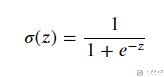1. # 手写 sigmoid 函数
2. def sigmoid(x):
3.     return 1 / (1 + np.exp(-x))
4. # 画图
5. matplotlib.style.use('classic')
6. x = np.linspace(-10, 10, 500)
7. plt.plot(x, sigmoid(x), 'b')
8. plt.grid(color='black', linestyle='--')
9. plt.xticks(np.arange(-10,10,2))
10. plt.yticks([0, 0.5, 1])
11. plt.ylim(0, 1)
12. plt.xlim(-10, 10)
13. plt.show()

Sigmoid函数的图像如下图所示: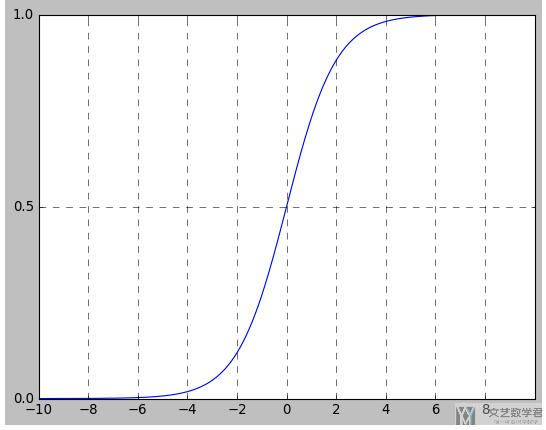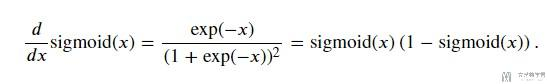• 当x=0的时候, 梯度达到最大, 此时是0.25.
• 当x向0的左右两边移动的时候, 梯度减小, 逐渐变为0.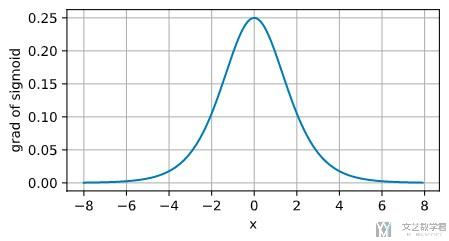### Tanh 函数

Tanh是双曲函数中的双曲正切函数, 他的数学公式如下所示, 他可以将数据压缩到[-1,1]的范围里: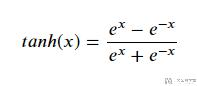1. def tanh(x):
2.     return (np.exp(x) - np.exp(-x))/(np.exp(x) + np.exp(-x))
3. x = np.linspace(-10, 10, 100)
4. plt.plot(x, tanh(x), 'b')
5. plt.grid(color='black', linestyle='--')
6. plt.xlabel('X Axis')
7. plt.ylabel('Y Axis')
8. plt.xticks(np.arange(-10,10,2))
9. plt.yticks([-1, 0, 1])
10. plt.ylim(-1, 1)
11. plt.xlim(-10, 10)
12. plt.show()

Tanh的函数图像如下所示: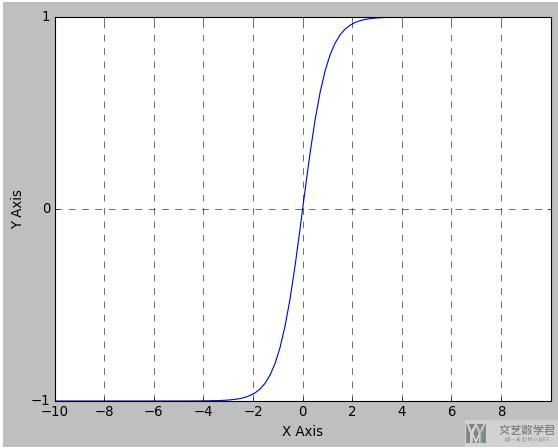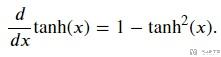• 在x=0的地方, tanh的导数达到最大, 此时是1;
• 在0的周围, 导数逐渐减小;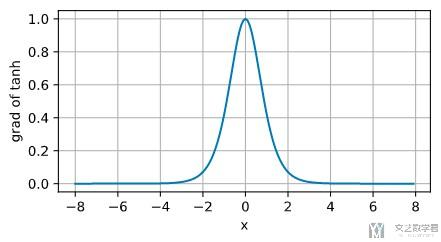### ReLU函数

Tanh(双曲正切函数)和Sigmoid函数相似, 也存在着梯度消失现象. 且由于解析式中存在幂运算, 计算起来较复杂. 因此, 为了解决梯度消失的问题, 线性修正单元函数(Rectified Linear Units，简称ReLU)孕育而生. ReLU是目前比较常用的激活函数之一. ReLU公式如下所示: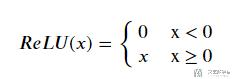接着我们绘制出ReLU的函数图像:
1. def relu(x):
2.     return np.where(x >= 0, x, 0)
3. y = np.linspace(-3, 3, 100)
4. plt.plot(y, relu(y), 'b')
5. plt.grid(linestyle='--')
6. plt.xticks(np.arange(-3,4,1))
7. plt.yticks([0, 1, 2, 3])
8. plt.ylim(0, 3)
9. plt.xlim(-3, 3)
10. plt.show()
ReLU的图像为: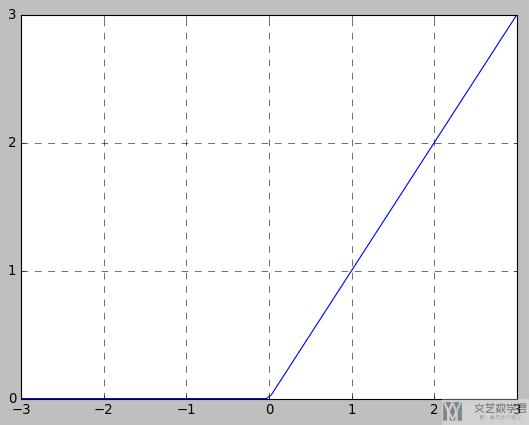接着, 我们绘制出ReLU的梯度, 可以看到他的梯度表现是很好的, 减轻了梯度消失的问题. 下面是绘制梯度的代码, 因为我们要绘制每个x的梯度值, 所以我们在反向传播的时候需要传入一个相同大小的向量, 相当于进行求和操作.
1. x = torch.arange(-8.0, 8.0, 0.1, requires_grad=True)
2. y = torch.relu(x)
3. y.backward(torch.ones_like(x), retain_graph=True)

## 关于梯度爆炸与梯度消失

### 梯度消失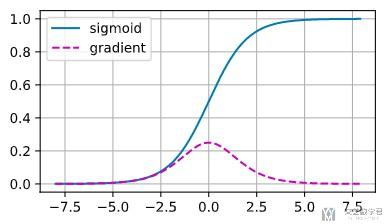### 梯度爆炸

1. M = torch.normal(0, 1, size=(4,4))
2. print('A single matrix \n',M)
3. """
4. A single matrix
5.  tensor([[-1.1204, -0.3560, -1.4320, -1.4257],
6.         [ 0.3308,  1.4008,  0.8356, -0.5116],
7.         [ 0.7456, -2.1095,  1.0507,  0.1431],
8.         [-0.5759, -0.1031,  0.4025,  0.8042]])
9. """
10. for i in range(100):
11.     M = torch.mm(M,torch.normal(0, 1, size=(4,4)))
12. print('After multiplying 100 matrices\n',M)
13. """
14. After multiplying 100 matrices
15.  tensor([[ 1.3603e+23, -9.0811e+22,  4.0481e+22, -8.3660e+21],
16.         [ 1.1984e+22, -8.0002e+21,  3.5663e+21, -7.3703e+20],
17.         [-9.9248e+22,  6.6257e+22, -2.9536e+22,  6.1040e+21],
18.         [ 1.4169e+22, -9.4589e+21,  4.2165e+21, -8.7141e+20]])
19. """

• 微信公众号
• 关注微信公众号
•• QQ群
• 我们的QQ群号
•• 本文由 发表于 2020年10月7日07:14:34
• 转载请务必保留本文链接：https://mathpretty.com/12518.html